# Practice Test: Polynomials

## 15 Questions MCQ Test Online MCQ Tests for Class 10 | Practice Test: Polynomials

Description
Attempt Practice Test: Polynomials | 15 questions in 20 minutes | Mock test for Class 10 preparation | Free important questions MCQ to study Online MCQ Tests for Class 10 for Class 10 Exam | Download free PDF with solutions
QUESTION: 1

### The zeros of the quadratic polynomial x2 + ax + h, a,b > 0 are

Solution:

p(x)=x2+ax+b

Let one of the zero of the polynomial is a then the other zero will be −a.

Sum of zeros=a+(−a)= −a / 1

=>0= −a/1

=>a=0

and product of the zeros =a(−a)=b/1

=>−a2=b

=>b=−a2

=>b has to be negative.

QUESTION: 2

### If p(x) = ax2 + bx + c and a + b + c = 0, then one zero is

Solution: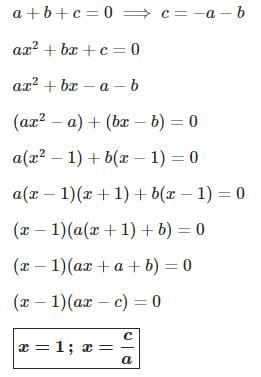QUESTION: 3

### Given that one of the zeroes of the cubic polynomial ax3 + bx2 + cx + d is zero, the product of the other two zeros is

Solution:

Let p(x) =ax3 + bx2 + cx + d

Given that, one of the zeroes of the cubic polynomial p(x) is zero.

Let α, β and γ are the zeroes of cubic polynomial p(x), where α = 0.

We know that,

Step-by-step explanation:

sum of two zeros at a time = c/a

∴αβ + βy + yα = c/a

∴0×β + βy + y×0 = c/a

βy = c/a

hence, product of 2 zeros = c/a

QUESTION: 4

If p(x) = ax2 + bx + c and a + c = b, then one of the zeroes is

Solution: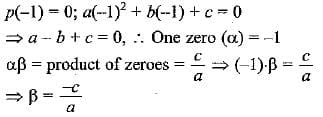QUESTION: 5

If one of the zeros of the quadratic polynomial  (k - 1) x2 + k x + 1 is - 3, then the value of k is

Solution:
QUESTION: 6

The number of polynomials haying zeroes as -2 and 5 is

Solution: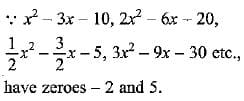QUESTION: 7

If one of the zeros  of the cubic polynomial x3 + ax2 + bx + c is - 1, then the product of the other two zeroes is

Solution:
QUESTION: 8

Given that one of the zeroes of the cubic polynomial ax3 + bx2 + cx + d is zero, the product of the other two zeroes is

Solution:

Let p(x) =ax3 + bx2 + cx + d
Given that, one of the zeroes of the cubic polynomial p(x) is zero.
Let α, β and γ are the zeroes of cubic polynomial p(x), where a = 0.
We know that,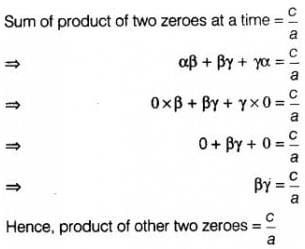QUESTION: 9

Given that two of the zeros of the cubic polynomial ax3 + bx2 + cx + d are 0, the value of c is

Solution:
QUESTION: 10

If 4x2 - 6x - m is divisible by x - 3, the value of m is exact divisor of

Solution:

Here p(3) = 0
⇒ 4(3)2 - 6 x 3 - m = 0
⇒ 36 - 18 - m = 0 ⇒ m =18
∴ Value of m is exactly divisible by 9.

QUESTION: 11

A quadratic polynomial, whose zeros are 5 and - 8 is

Solution:
QUESTION: 12

Which one of the following statements is correct

Solution: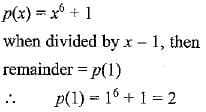QUESTION: 13

The zeros of the quadratic polynomial x2 + kx + k, k ≠ 0

Solution:
QUESTION: 14

Consider the following statements
(i) x - 2 is a factor of x3 - 3x2 + 4x - 4.
(ii) x + 1 is a factor of 2x3 + 4x + 6
(iii) x - 1 is a factor of x5 + x4 - x3 + x2 - x + 1

In these statements

Solution:

x - 2 is a factor of x3 - 3x2 + 4x - 4
∵ remainder is zero
Similarly x + 1 is a factor of 2x3 + 4x + 6
but x - 1 is not a factor of x5 + x4 - x3 + x2 - x + 1
∵ remainder is not zero
∴ Statements 1 and 2 are correct.

QUESTION: 15

The degree of the remainder r(x) when p (x) = bx3 + cx + d is divided by a polynomial of degree 4 is ​

Solution:Use Code STAYHOME200 and get INR 200 additional OFF Use Coupon Code